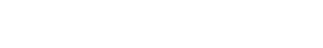# Walkthrough of Acid-Base reactions (4) – pKa

So last time we went through all the different trends that affect acidity. The bottom line is that any factor which stabilizes the conjugate base will result in increased acidity.

Now it’s fine to know trends – it’s extremely important, in fact – but what do you do when you want to compare the acidity of two molecules that aren’t connected by a trend? For instance, resonance increases the acidity of alcohols, but by how much? More than you’d increase acidity by changing OH to SH? What if you added an electron withdrawing group?

It’s impossible to sort out all the opposing variables this way. Trends are only qualitative guides to this topic.

In order to answer this question we’ll need some hard data, and there’s only one way to get it in organic chemistry – through measurement.

Let’s look at hydroiodic acid,  H–I. In solution (let’s use water)  H–I will protonate water to give H3O(+) and I(-). The reverse reaction also operates. [I (–) reacts with H3O(+) to give H-I back]. So this reaction is an equilibrium.Here’s the useful part. We can measure the equilibrium constant of this reaction, and that will tell us about the relative acidity of H-I. The specifics of how we can measure this are another story, but for our purposes, let me assure you that these types of equilibrium constants can be measured accurately.

For H-I, it turns out that the equilibrium constant for this reaction is about 10,000,000,000. That means for every molecule of HI, there are 10 billion molecules of I(-). In other words, H-I donates its proton very readily to give its conjugate base, which is a prime example of a strong acid.So what? Well, we can do this not just for H-I, but for ANY species with a hydrogen. This equilibrium is the “ruler” by which we can measure any acid – by its equilibrium constant.*

We call this equilibrium constant Ka – the “acidity constant”.

Let’s look at 3 examples. Note that these are somewhat oversimplified in that the solvent has been left out.*Now comparing acidity by numbers with lots of exponents after them is not the most convenient way to do things. So instead, we’ve taken to using a logarithmic scale. These are common – the Richter scale is logarithmic, for instance – an 8.0 magnitude quake is 10 times more powerful than a 7.0 magnitude quake.

For acidity, the number we use is called “pKa”. It’s obtained by taking the logarithm of the acidity constant Ka, and we arbitrarily decide to multiply it by negative one [the vast majority of Ka values are less than 1, and we’re generally more comfortable dealing with positive numbers].So for H-I, the pKa is -10 (representing a very strong acid), that for methanol is 15 (a weak acid), and for methane, it’s 50 (an extremely weak acid).

That scale comprises 60 orders of magnitude. That’s a huge number!!!!!

To give you an idea of the scale, of pKa, this is the range of the smallest value for length (the Planck length, 10 to the -35 m), to the width of the known universe (~10 to the 26 meters). This website has a phenomenal animation of this.

Anyhow, these measurements have been done for thousands of different molecules now. The result is a big table that allows us to compare the acidity of all kinds of different functional groups. Here’s an example of a pKa table from a previous post.The pKa table is your friend. In one document, it gives you information on the scope and magnitude of a wide range of chemical behavior – the strongest of the strong acids, and the weakest of the weak acids. And since the stronger the acid, the weaker the conjugate base, it also provides information about basicity.

Next Post: How to Use A pKa Table

* N.B. The choice of solvent for these measurements is important due to the “leveling effect”. For instance  Water is commonly used as a solvent for molecules with a pKa value less than zero and no greater than about 15, since there can be no stronger acid in water than H3O(+) and no stronger base than HO(-). To get some of the more extreme values other solvents must be used, such as dimethyl sulfoxide (DMSO). If you end up in a more advanced organic chemistry course, look at some of these values with a harder eye through that lens. Strongly recommended – Evans’ pKa table gives values in H2O and DMSO. And there is the heroic efforts of Hans Reich at Wisconsin in compiling pKa values.